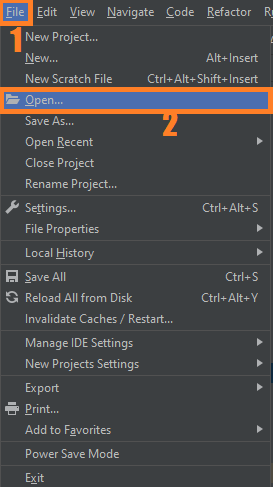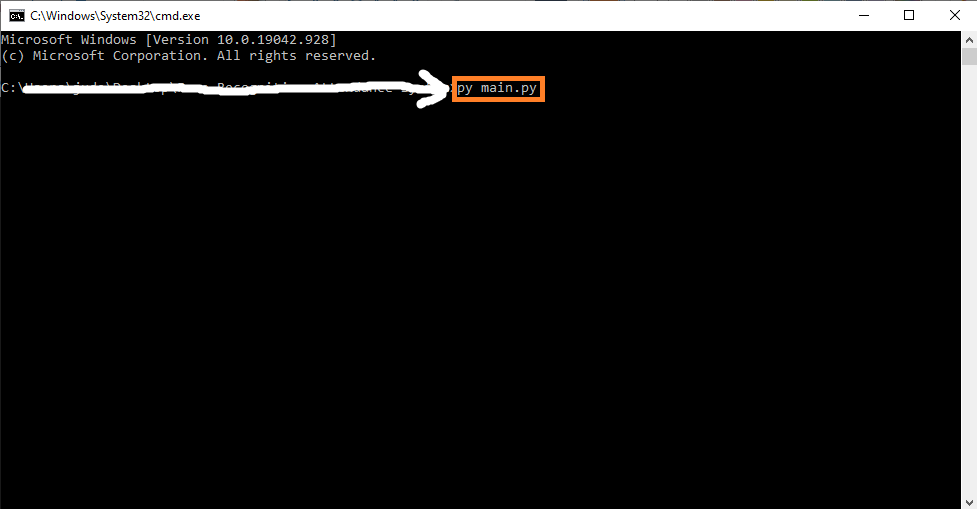# Real-Time Emotion Detection OpenCV Python With Source Code

## Real-Time Emotion Detection OpenCV Python With Source Code

The Real-Time Emotion Detection OpenCV Python was developed using Python OpenCV, Emotion Detection or Facial Expression Classification is a widely researched topic in today’s Deep Learning arena. To classify your emotions in real-time using just you camera and some lines of code is actually a big step towards Advanced Human Computer interaction.

A Emotion Detection OpenCV Python Detecting the real-time emotion of the person with a camera input is one of the advanced features in the machine learning process. The detection of emotion of a person using a camera is useful for various research and analytics purposes.

The detection of emotion is made by using the machine learning concept. You can use the trained dataset to detect the emotion of the human being. For detecting the different emotions, first, you need to train those different emotions, or you can use a dataset already available on the internet. In this article, we will discuss creating a Python program to detect the real-time emotion of a human being using the camera.

By the way if you are new to python programming and you don’t know what would be the the Python IDE to use, I have here a list of Best Python IDE for Windows, Linux, Mac OS that will suit for you. I also have here How to Download and Install Latest Version of Python on Windows.

To start executing Real-Time Emotion Detection OpenCV Python With Source Code, make sure that you have installed Python 3.9 and PyCharm in your computer.

## Real-Time Emotion Detection OpenCV Python With Source Code : Steps on how to run the project

Time needed: 5 minutes

These are the steps on how to run Real-Time Emotion Detection OpenCV Python With Source Code

First, download the given source code below and unzip the source code.• Step 2: Import the project to your PyCharm IDE.• Step 3: Run the project.

last, run the project with the command “py main.py”## Installed Libraries

```import cv2.cv2 as cv2
import numpy as np```

## Complete Source Code

```import cv2.cv2 as cv2
import numpy as np

from utils.image_classifier import ImageClassifier, NO_FACE_LABEL

# Color RGB Codes & Font
WHITE_COLOR = (255, 255, 255)
GREEN_COLOR = (0, 255, 0)
BLUE_COLOR = (255, 255, 104)
FONT = cv2.QT_FONT_NORMAL

# Frame Width & Height
FRAME_WIDTH = 640
FRAME_HEIGHT = 490

class BoundingBox:
def __init__(self, x, y, w, h):
self.x = x
self.y = y
self.w = w
self.h = h

@property
def origin(self) -> tuple:
return self.x, self.y

@property
def top_right(self) -> int:
return self.x + self.w

@property
def bottom_left(self) -> int:
return self.y + self.h

def draw_face_rectangle(bb: BoundingBox, img, color=BLUE_COLOR):
cv2.rectangle(img, bb.origin, (bb.top_right, bb.bottom_left), color, 2)

def draw_landmark_points(points: np.ndarray, img, color=WHITE_COLOR):
if points is None:
return None
for (x, y) in points:
cv2.circle(img, (x, y), 1, color, -1)

def write_label(x: int, y: int, label: str, img, color=BLUE_COLOR):
if label == NO_FACE_LABEL:
cv2.putText(img, label.upper(), (int(FRAME_WIDTH / 2), int(FRAME_HEIGHT / 2)), FONT, 1, color, 2, cv2.LINE_AA)
cv2.putText(img, label, (x + 10, y - 10), FONT, 1, color, 2, cv2.LINE_AA)

class RealTimeEmotionDetector:
CLAHE = cv2.createCLAHE(clipLimit=2.0, tileGridSize=(8, 8))

vidCapture = None

def __init__(self, classifier_model: ImageClassifier):
self.__init_video_capture(camera_idx=0, frame_w=FRAME_WIDTH, frame_h=FRAME_HEIGHT)
self.classifier = classifier_model

def __init_video_capture(self, camera_idx: int, frame_w: int, frame_h: int):
self.vidCapture = cv2.VideoCapture(camera_idx)
self.vidCapture.set(cv2.CAP_PROP_FRAME_WIDTH, frame_w)
self.vidCapture.set(cv2.CAP_PROP_FRAME_HEIGHT, frame_h)

return frame

def transform_img(self, img: np.ndarray) -> np.ndarray:
# load the input image, resize it, and convert it to gray-scale
gray_img = cv2.cvtColor(img, cv2.COLOR_BGR2GRAY)  # convert to gray-scale
resized_img = self.CLAHE.apply(gray_img)  # resize
return resized_img

def execute(self, wait_key_delay=33, quit_key='q', frame_period_s=0.75):
frame_cnt = 0
predicted_labels = ''
old_txt = None
rectangles = [(0, 0, 0, 0)]
landmark_points_list = [[(0, 0)]]
while cv2.waitKey(delay=wait_key_delay) != ord(quit_key):
frame_cnt += 1

if frame_cnt % (frame_period_s * 100) == 0:
frame_cnt = 0
predicted_labels = self.classifier.classify(img=self.transform_img(img=frame))
rectangles = self.classifier.extract_face_rectangle(img=frame)
landmark_points_list = self.classifier.extract_landmark_points(img=frame)
for lbl, rectangle, lm_points in zip(predicted_labels, rectangles, landmark_points_list):
draw_face_rectangle(BoundingBox(*rectangle), frame)
draw_landmark_points(points=lm_points, img=frame)
write_label(rectangle, rectangle, label=lbl, img=frame)

if old_txt != predicted_labels:
print('[INFO] Predicted Labels:', predicted_labels)
old_txt = predicted_labels

cv2.imshow('Emotion Detection - Mimics', frame)

cv2.destroyAllWindows()
self.vidCapture.release()

def run_real_time_emotion_detector(
classifier_algorithm: str,
predictor_path: str,
dataset_csv: str,
dataset_images_dir: str = None):
from utils.data_land_marker import LandMarker
from utils.image_classifier import ImageClassifier
from os.path import isfile

land_marker = LandMarker(landmark_predictor_path=predictor_path)

if not isfile(dataset_csv):  # If data-set not built before.
from data_preparer import run_data_preparer
run_data_preparer(land_marker, dataset_images_dir, dataset_csv)
else:
print('[INFO]', f'Dataset file: "{dataset_csv}" found.')

classifier = ImageClassifier(csv_path=dataset_csv, algorithm=classifier_algorithm, land_marker=land_marker)
print('[INFO] Opening camera, press "q" to exit..')
RealTimeEmotionDetector(classifier_model=classifier).execute()

if __name__ == "__main__":
"""The value of the parameters can change depending on the case."""
run_real_time_emotion_detector(
classifier_algorithm='RandomForest',  # Alternatively 'SVM'.
predictor_path='utils/shape_predictor_68_face_landmarks.dat',
dataset_csv='data/csv/dataset.csv',
dataset_images_dir='data/raw'
)
print('Successfully terminated.')
```

## Summary

Detecting the real-time emotion of the person with a camera input is one of the advanced features in the machine learning process. The detection of emotion of a person using a camera is useful for various research and analytics purposes.

The detection of emotion is made by using the machine learning concept. You can use the trained dataset to detect the emotion of the human being. For detecting the different emotions, first, you need to train those different emotions, or you can use a dataset already available on the internet. In this article, we will discuss creating a Python program to detect the real-time emotion of a human being using the camera.

## Inquiries

### 8 thoughts on “Real-Time Emotion Detection OpenCV Python With Source Code”

1.how to import this project??

•For the importing you can watch in the you tube on how to import.

2.which video we can watch?

3.sir after copy the libraries from the top what you diid in the main function can you tell me that because after running the command prompt its showing first line error.

•What is the error

4.can i have your presentation and report details

•So far we don’t have the report or documentation for this project

5.Where did you take the dataset? Did you make it or did you take it somewhere? Please post the source.